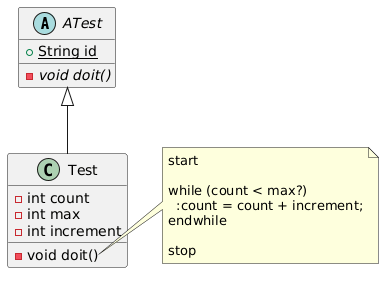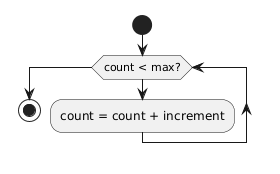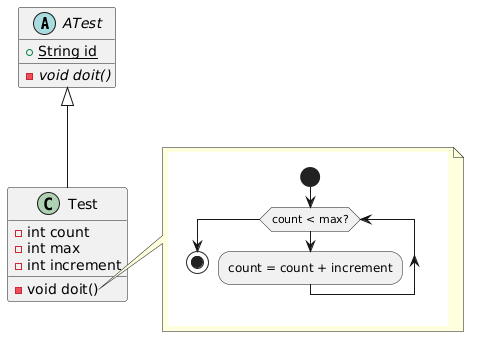# embed sub diagrams in diagram like a note

asked Jan 8, 2018

I want to be able to write something like this

@startuml

abstract ATest {
{static} +String id
{abstract} -void doit()
}

class Test {
-int count
-int max
-int increment
-void doit()
}

ATest <|-- Test

subdiagram right of  Test::doit
start

while (count < max?)
:count = count + increment;
endwhile

stop
end subdiagram
@enduml

such that it would draw something likebut with the activity diagramin the note rather than the code to define the activity diagram.

I would like this to work for any set of diagrams (in the start/end(uml) block) with unlimited levels of nesting, but am currently only looking to embed activity diagrams in class diagrams.

+1 vote
answered Jan 26, 2018 by (7,810 points)

You can do this:

```@startuml
abstract ATest {
{static} +String id
{abstract} -void doit()
}

class Test {
-int count
-int max
-int increment
-void doit()
}

ATest <|-- Test

note right of Test::doit()
{{
start

while (count < max?)
:count = count + increment;
endwhile

stop
}}
end note
@enduml```you may also embed a diagram as an image.